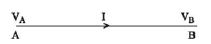Physics

# Explain Ohm’s Law

The current passing through a conductor at constant temperature is directly proportional to the potential difference between the two ends of the conductor.

By proportionality it means- if the potential difference between the two ends is doubled, the current flowing through the conductor will be doubled. Again, if the potential difference between the two terminals is made one third, the current passing through the conductor will be one third.

Assume AB is a conducting wire. The potential of its two terminals are VA and VB [Figure] respectively. If VA > VB, The potential difference between the two terminals of the conductor will be V = VA – VB.Now at constant temperature, if the current passing through the conductor is I, then according to ohm’s law,

I ∞ V

= V/I = R = Constant

This constant is called the resistance of the conductor at that temperature.

Or, I = V/R